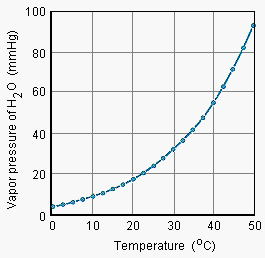Liquids

Liquids

The Structure of Liquids

The difference between the structures of gases, liquids, and solids can be best understood by comparing the densities of substances that can exist in all three phases. As shown in the table below, the density of a typical solid is about 20% larger than the corresponding liquid, while the liquid is roughly 800 times as dense as the gas.

Densities of Solid, Liquid, and Gaseous Forms of Three Elements

 Solid (g/cm3) Liquid (g/cm3) Gas (g/cm3) Ar 1.65 1.40 0.001784 N2 1.026 0.8081 0.001251 O2 1.426 1.149 0.001429

The figure below shows a model for the structure of a liquid that is consistent with these data.The key points of this model are summarized below.

• The particles that form a liquid are relatively close together, but not as close together as the particles in the corresponding solid.
• The particles in a liquid have more kinetic energy than the particles in the corresponding solid.
• As a result, the particles in a liquid move faster in terms of vibration, rotation, and translation.
• Because they are moving faster, the particles in the liquid occupy more space, and the liquid is less dense than the corresponding solid.
• Differences in kinetic energy alone cannot explain the relative densities of liquids and solids. This model therefore assumes that there are small, particle-sized holes randomly distributed through the liquid.
• Particles that are close to one of these holes behave in much the same way as particles in a gas, those that are far from a hole act more like the particles in a solid.What Kinds of Materials Form Liquids at Room Temperature?

Three factors determine whether a substance is a gas, a liquid, or a solid at room temperature and atmospheric pressure:

 (1) the strength of the bonds between the particles that form the substance (2) the atomic or molecular weight of these particles (3) the shape of these particles

When the force of attraction between the particles are relatively weak, the substance is likely to be a gas at room temperature. When the force of attraction is strong, it is more likely to be a solid. As might be expected, a substance is a liquid at room temperature when the intermolecular forces are neither too strong nor too weak. The role of atomic or molecular weights in determining the state of a substance at room temperature can be understood in terms of the kinetic molecular theory, which includes the following assumption: The average kinetic energy of a collection of gas particles depends on the temperature of the gas, and nothing else. This means that the average velocity at which different molecules move at the same temperature is inversely proportional to the square root of their molecular weights.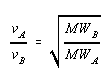Relatively light molecules move so rapidly at room temperature they can easily break the bonds that hold them together in a liquid or solid. Heavier molecules must be heated to a higher temperature before they can move fast enough to escape from the liquid. They therefore tend to have higher boiling points and are more likely to be liquids at room temperature.

The relationship between the molecular weight of a compound and its boiling point is shown in the table below. The compounds in this table all have the same generic formula: CnH2n+2. The only difference between these compounds is their size and therefore their molecular weights.

Melting Points and Boiling Points of Compounds with the Generic Formula CnH2n+2

 Compound Melting Point (oC) Boiling Point (oC) CH4 -182 -164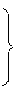C2H6 -183.3 -88.6 C3H8 -189.7 -42.1 C4H10 -138.4 -0.5 C5H12 -130 36.1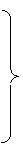C6H14 -95 69 C7H16 -90.6 98.4 C8H18 -56.8 125.7 C9H20 -51 150.8 C10H22 -29.7 174.1
Gases at room temperature
liquids at room temperature

As shown by the figure below, the relationship between the molecular weights of these compounds and their boiling points is not a straight line, but it is a remarkably smooth curve.The data in the figure below show how the shape of a molecule influences the melting point and boiling point of a compound and therefore the probability that the compound is a liquid at room temperature.

 Compound Melting Point (oC) Boiling Point (oC)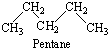-130 36.1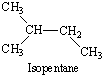-159.9 27.8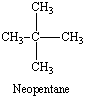-16.5 9.5

The three compounds in this figure are isomers (literally, "equal parts"). They all have the same chemical formula, but different structures. One of these isomersneopentaneis a very symmetrical molecule with four identical CH3 groups arranged in a tetrahedral pattern around a central carbon atom. This molecule is so symmetrical that it easily packs to form a solid. Neopentane therefore has to be cooled to only -16.5oC before it crystallizes.

Pentane and isopentane molecules have zigzag structures, which differ only in terms of whether the chain of C-C bonds is linear or branched. These less symmetrical molecules are harder to pack to form a solid, so these compounds must be cooled to much lower temperatures before they become solids. Pentane freezes at -130oC. Isopentane must be cooled to almost -160oC before it forms a solid.

The shape of the molecule also influences the boiling point. The symmetrical neopentane molecules escape from the liquid the way marbles might pop out of a box when it is shaken vigorously. The pentane and isopentane molecules tend to get tangled, like coat hangers, and must be heated to higher temperatures before they can boil. Unsymmetrical molecules therefore tend to be liquids over a larger range of temperatures than molecules that are symmetrical.Vapor Pressure

A liquid doesn't have to be heated to its boiling point before it can become a gas. Water, for example, evaporates from an open container at room temperature (20oC), even though the boiling point of water is 100oC. We can explain this with the diagram in the figure below. The temperature of a system depends on the average kinetic energy of its particles. The term average is in this statement because there is an enormous range of kinetic energies for these particles.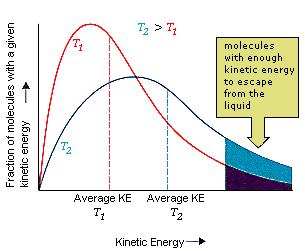Even at temperatures well below the boiling point of a liquid, some of the particles are moving fast enough to escape from the liquid.

When this happens, the average kinetic energy of the liquid decreases. As a result, the liquid becomes cooler. It therefore absorbs energy from its surroundings until it returns to thermal equilibrium. But as soon as this happens, some of the water molecules once again have enough energy to escape from the liquid. In an open container, this process continues until all of the water evaporates.

In a closed container some of the molecules escape from the surface of the liquid to form a gas as shown in the figure below. Eventually the rate at which the liquid evaporates to form a gas becomes equal to the rate at which the gas condenses to form the liquid. At this point, the system is said to be in equilibrium (from the Latin, "a state of balance"). The space above the liquid is saturated with water vapor, and no more water evaporates.The vapor pressure of a liquid is literally the pressure of the gas (or vapor) that collects above the liquid in a closed container at a given temperature.

The pressure of the water vapor in a closed container at equilibrium is called the vapor pressure. The kinetic molecular theory suggests that the vapor pressure of a liquid depends on its temperature. As can be seen in the graph of kinetic energy versus number of molecules, the fraction of the molecules that have enough energy to escape from a liquid increases with the temperature of the liquid. As a result, the vapor pressure of a liquid also increases with temperature.

The figure below shows that the relationship between vapor pressure and temperature is not linearthe vapor pressure of water increases more rapidly than the temperature of the system.